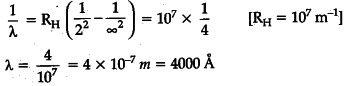# Define ionization energy.How would the ionization energy

Define ionization energy.How would the ionization energy change when electron in hydrogen atom is replaced by a particle of mass 200 times that of the electron but having the same charge ?
OR
Calculate the shortest wavelength of the spectral lines emitted in Balmer series.
[Given Rydberg constant, R = ${ 10 }^{ 7 }$ m-1]

Ionisation energy is the minimum amount of energy required to remove an electron from the outermost orbit of a neutral atom in it’s ground state.
OR
The shortest wavelength of spectral lines emitted in Balmer series —
Series limit of Balmer series corresponds to n=\infty It has maximum energy and hence shortest wavelength —